Technical Information Site of Power Supply Design

• Basic Knowledge
• AC/DC
• Design Method of PWM AC/DC Flyback Converters
• Designing Isolated Flyback Converter Circuits: Transformer Design (Calculating numerical values)

2016.04.21 AC/DC

# Designing Isolated Flyback Converter Circuits: Transformer Design (Calculating numerical values)

Design Method of PWM AC/DC Flyback Converters

Of the required transformer design steps for a flyback converter, we begin with the calculation of the numerical values necessary for the design of the transformer, based on power supply specifications. Basically, calculations are made according to the equations provided for each parameter. For your reference, relevant transformer design information is provided in the BM1P061FJ Application Notes and other documents for the IC1 to be used in the design task. In this section, for ease of understanding the parts to be explained are shown in enlarged views. For the structure of the entire circuit, see the section on [Design Example Circuits].

The circuit diagram shown below represents excerpts from the transformer T1 part of the example circuit. In addition to the input primary winding Np and output secondary winding Ns, the transformer T1 includes a winding Nd that generates VCC voltage for the IC1.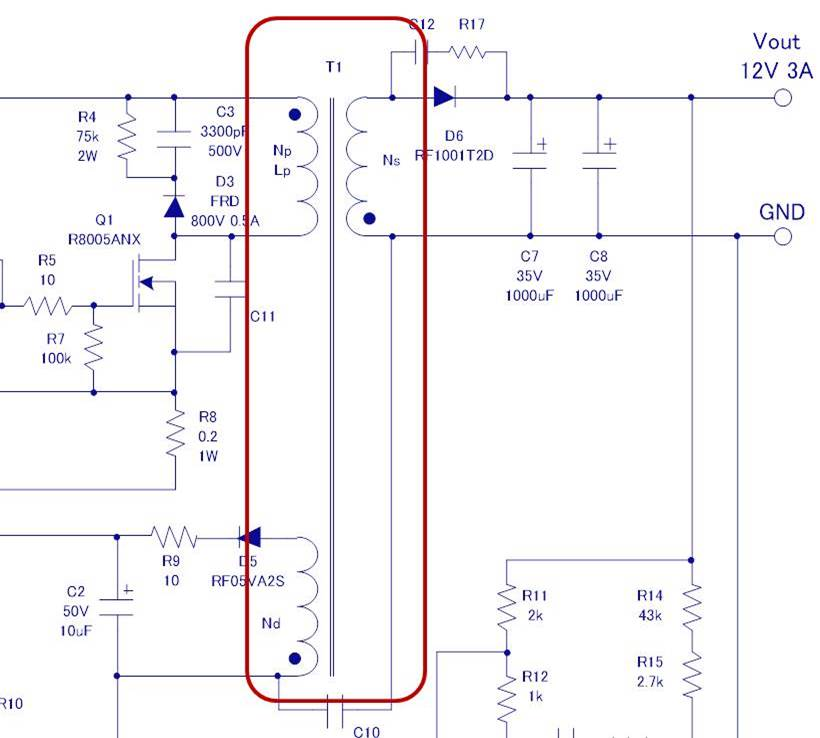Transformer T1 design procedure
The listed items below describe the procedure for designing a transformer T1. In the following procedure, you calculate numerical values and derive parameters for the transformer listed in the table below. For windings and symbols for the electric current that flows, see the transformer schematic diagram provided in the lower right area below.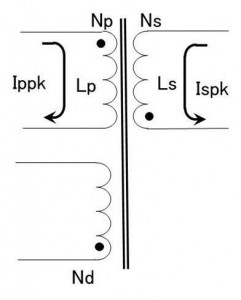The values derived as transformer T1 parameters

Core Size Inductance Number of turns Number of turns Number of turns

(1) Setting a flyback voltage VOR

The flyback voltage VOR is equal to VO (the secondary Vout plus the VF for the secondary diode D6) multiplied by the transformer winding ratio Np:Ns. Setting the flyback voltage VOR determines the winding ratio Np：Ns and the Duty ratio. The basic equation and an example are given below.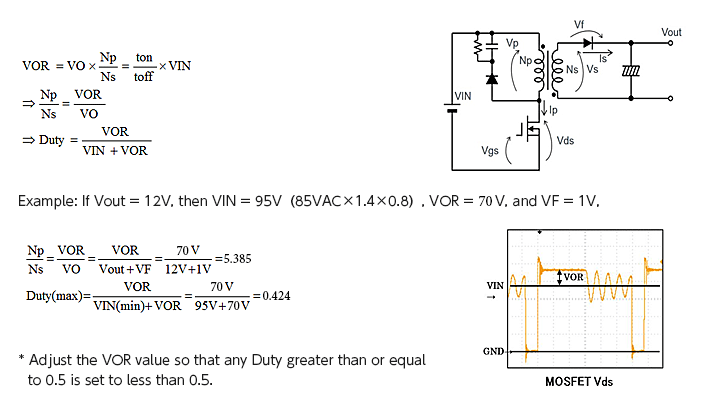In the example, the winding ratio Np：Ns turns out to be 5.385, and the Duty (max) is 0.424. Empirically, a desirable Duty (max) value is 0.5 or less. If the calculation indicates a Duty value greater than 0.5, the VOR should be adjusted.

In terms of the operating principles of the flyback converter, we chose as a starting point the setting of the flyback voltage VOR in order to clearly identify the Vds of the switching transistor that is applied to the primary winding, that is, the quantity VIN + VOR. In another approach, it is possible to use the maximum Duty ratio as a starting point.

For details on the flyback circuit operation and the voltages, refer to “PWM Flyback Converter Operation (Continuous mode)” in “Flyback Converter Basic Circuit and Characteristics

(2) Calculating the secondary winding inductance Ls and secondary-side peak current
Ispk

In succession, we calculate the secondary-side winding inductance Ls and the secondary-side peak current Ispk. The equations given below represent conditions for the discontinuous mode which is a condition for the example circuit, such that where equality represents a critical point (a bifurcation point between the continuous and discontinuous modes). The critical point should be reached when the load current is equal to Iomax.

To provide for a margin, such as an over-load protection point, the maximum load current should be 1.2 times the Iout. Since specifications for Iout are 3A, Iomax should be 3.6A. In terms of specifications, Vout should be equal to 12V, and the VF and Duty, values calculated in Step (1) should be used.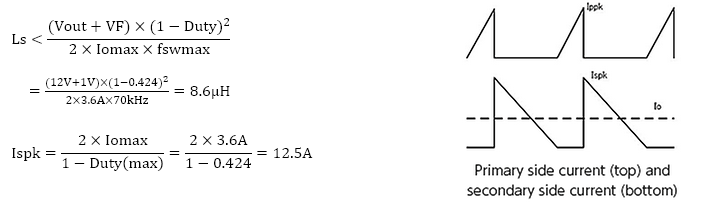From the above equations, the primary side winding inductance Ls=8.6μH and the secondary side peak current Ispk = 12.5A were calculated. For your reference, primary and secondary current waveforms are shown in the above drawings.

(3) Calculating the primary winding inductance Lp and primary peak current Ippk

In the next step, based on the equations given below and using the above calculation results, we obtain the primary winding inductance Lp and the primary peak current Ippk: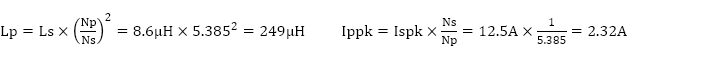where the calculated Lp represents one of the values that are derived as parameters for the transformer T1.

(4) Determining the transformer size

The size of the transformer core is determined based upon the output power Po (W). The table below shows the relationship between general output power for a flyback converter and the required core size. Because the output power for this design example is Po=36W, we select the EER28 core size.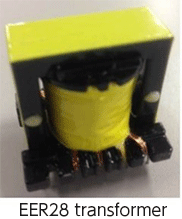Output power Po(W) Core size Core cross section Ae（mm2
～ 30 EI25/EE25 41
～ 60 EI28/EE28/EER28 84

* The above values only represent rough approximations. For details, transformer manufacturers should be consulted.

(5) Calculating the primary winding turns Np

The primary winding turns Np must be set initially so that the magnetic flux density will fall within the tolerance range. Since the maximum magnetic flux density B (T) for the commonly available ferrite core is 0.4Tat 100℃, by setting Bsat = 0.35T and substituting into Lp and Ippk, we obtain the primary winding turns Np: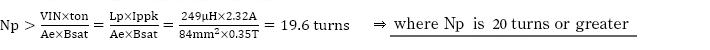In the next step, to prevent occurrence of any magnetic saturation, we set Np from the AL-Value-NI properties. In performing this step, the Bsat condition formula must be satisfied.

If AL-Value=280nH/turns2,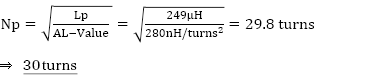This means that if Lp is 249μH, the AL-Value for 30 turns is 249μH/302≒276.7nH/turns2.

The NI value can be determined from the following equation: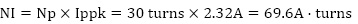Now that AL-Value and NI have been determined, from the AL-Value-NI characteristic graph for the EER28 core size, we confirm that the values are within the tolerance range. If they are out of range, we adjust the value of Np.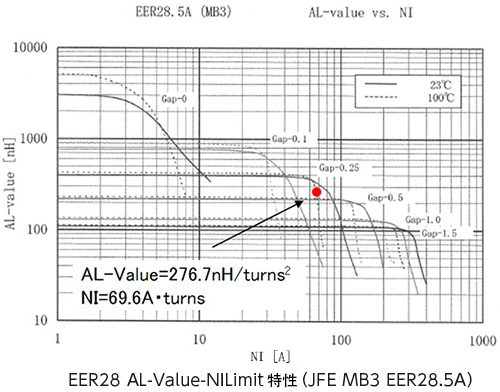(6) Calculating the secondary winding turns Ns

After calculating the primary winding turns, we calculate the secondary winding count Ns. Since we have already determined that the primary winding turns Np is 34 turns and the Np:Ns ratio is 5:1, we substitute these values into the following equations: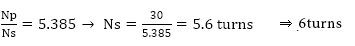(7) Calculating the VCC winding turns Nd

Finally, we calculate the winding turns necessary to generate the VCC for IC1:

Since VCC is 15V, through the diode D6 based on the number of turns, if the VF for the diode, VF_vcc is 1V,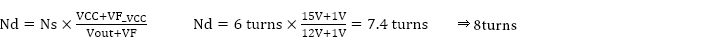This concludes the calculations of numerical values that determine the specifications for the transformer. By substituting the calculated values into the table of specifications that was shown at the beginning, we proceed with the structural design step.

Core JFE MB3 EER28.5A or compatible 249 μH 30 turns 6 turns 8 turns

Although the above equations, numerous at a glance, may look intimidating, they are relatively simple formulas; you should try using them. When overall specifications have been worked out, you can proceed with the transformer design task by utilizing the support available from the IC and transformer manufacturers.

#### Key Points:

・Basically, the design of a transformer that conforms to the circuit being designed will be necessary.

・Although some engineers may shy away from designing a transformer due to the tediousness
of the task, support available from IC and transformer manufacturers can be tapped into.